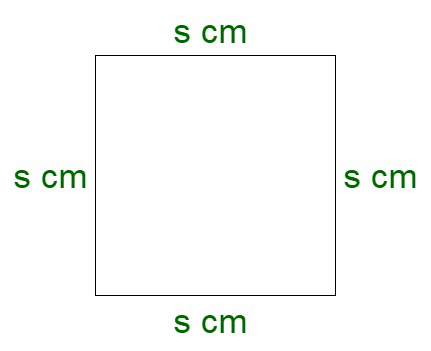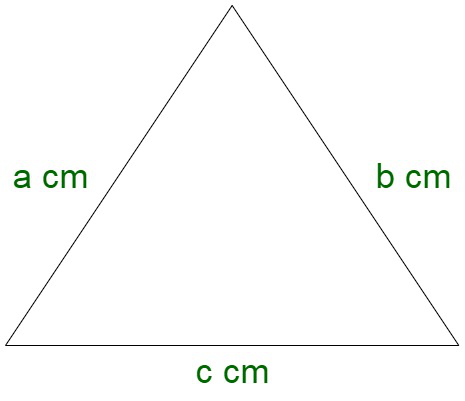# Can a triangle and a square have the same perimeter?

• Last Updated : 02 Nov, 2021

A square is a geometrical figure which is formed of four equal sides. It is a regular quadrilateral where all the angles are right angles. Basically, it can be considered as a special case of a rectangle, where all the sides are equal in length. For instance, a square courtyard.Properties of square

• All four sides of a square are equal.
• The diagonals of a square are equal.
• All the four angles of a square are equal.
• The diagonals of a square bisect its angles.

Perimeter of a square

The perimeter of a square is referred to as the total length of the boundary enclosing the geometrical figure.

Perimeter of square = Sum of all sides of a square

Let us assume s to be the side of the square.

Since, we know, all the sides of the square are equal.

Perimeter of square = s + s + s + s

Perimeter of square = 4s ….. (I)

Triangle

A triangle is a geometrical figure which is formed of three sides. It is a regular quadrilateral where all the angles may or may not be different. For instance, a triangular courtyard.Properties of triangle

• The sum of all the angles of a triangle is equal to 180°.
• Area of a triangle = ½ × Base × Height
• The sum of the length of the two sides of a triangle is greater than the length of the third side (By Pythagoras theorem)

Perimeter of a triangle

The perimeter of a triangle is referred to as the total length of the boundary enclosing the geometrical figure.

Perimeter of triangle = Sum of all sides of the triangle

Let us assume a, b and c to be the sides of the triangle.

Perimeter of triangle = a + b + c

Perimeter of triangle = a + b + c……. (II)

### Can a triangle and a square have the same perimeter?

Solution:

Since, we know,

Perimeter of square = 4s  …(By I)

Perimeter of triangle = a + b + c… (By II)

As per the question, we get, equating we get,

Perimeter of square = Perimeter of triangle

4s = a + b + c

On solving, we get,

=> s = (a + b + c)/ 4 …… III

Therefore, the corresponding perimeters can be equal when the side of the square is equal to 1/4th of the sum of sides of the triangle.

### Sample Questions

Question 1: What will be the side of the square when the sides of the triangle to be 2, 4, and 4 cm.

Solution:

Given,

The sides of the triangle to be 2, 4, and 4 cm.

Now the perimeter of the triangle is given by,

Perimeter of the triangle, P1 = sum of sides of the triangle

= 2 + 4 + 4 cm

= 10 cm

Now,

By the III formula, we have,

Since the perimeters of both the figures are equal,

Perimeter of triangle = Perimeter of a square

Let us assume s to be the side of the square.

⇒ 10 cm = 4 * s

⇒  s = 10/4 cm

⇒  s = 2.5 cm

Question 2: Assume a square and a triangle having the same perimeters. Then if the diagonal of the square is 24√2. So find the area of the triangle?

Solution:

As per the question

Perimeter of triangle = Perimeter of Square

We are given that

Diagonal of square = 24√2

Therefore,

Side of square is, Side =Side of square =Side of square = 24 cm

Perimeter of square = 4 × side

= 4 × 24

= 96 cm

As per the question

Perimeter of triangle = Perimeter of Square

Perimeter of triangle = 96

96 = 3 × side

Side =Side of triangle = 32

Further,

Area of triangle =Area of triangle = 256√3 cm2

Question 3: Assume that the perimeter of the square and triangle are the same. If one side of the equilateral triangle is 18 cm. Then find the perimeter of the equilateral triangle and the square?

Solution:

Here,

We have one side of the equilateral triangle 18 cm

we are given that the perimeter of the equilateral triangle and square are the same

Perimeter of Equilateral triangle = Side + Side + Side = 3a

Perimeter of Square = Side + Side + Side + Side = 4s

As per the question

Perimeter of Equilateral triangle = Perimeter of Square

3a = 4s

We have a = 18 cm

3 × 18 = 4 × s

54 = 4 × s

s =s = 13.5 cm

Therefore,

Perimeter of Equilateral triangle = 3a

= 3 × 18

= 54 cm

Perimeter of Square = 4s

= 4 × 13.5

= 54 cm

Question 4: What will be the side of the square when the sides of the triangle to be 4, 8, and 8 cm.

Solution:

Given,

The sides of the triangle to be 4, 8, and 8 cm.

Now the perimeter of the triangle is given by,

Perimeter of the triangle, P1 = sum of sides of the triangle

= 4 + 8 + 8 cm

= 20 cm

Now,

By the III formula, we have,

Since the perimeters of both the figures are equal,

Perimeter of triangle = Perimeter of a square

Let us assume s to be the side of the square.

⇒ 20 cm = 4 * s

⇒  s = 20/4 cm

⇒  s = 5 cm

Question 5: Assume that the perimeter of the square and triangle are the same. If one side of an equilateral triangle is 36 cm. Then find the perimeter of an equilateral triangle and the square?

Solution:

Here,

We have one side of the equilateral triangle 36 cm

we are given that the perimeter of the equilateral triangle and square are the same

Perimeter of Equilateral triangle = Side + Side + Side = 3a

Perimeter of Square = Side + Side + Side + Side = 4s

As per the question

Perimeter of Equilateral triangle = Perimeter of Square

3a = 4s

We have a = 36 cm

3 × 36 = 4 × s

108 = 4 × s

s =s = 27 cm

Therefore,

Perimeter of Equilateral triangle = 3a

= 3 × 36

= 108 cm

Perimeter of Square = 4s

= 4 × 27

= 108 cm

My Personal Notes arrow_drop_up